Courses

# Number System (Advance Level) - 1

## 15 Questions MCQ Test Question Bank for GATE Computer Science Engineering | Number System (Advance Level) - 1

Description
This mock test of Number System (Advance Level) - 1 for Computer Science Engineering (CSE) helps you for every Computer Science Engineering (CSE) entrance exam. This contains 15 Multiple Choice Questions for Computer Science Engineering (CSE) Number System (Advance Level) - 1 (mcq) to study with solutions a complete question bank. The solved questions answers in this Number System (Advance Level) - 1 quiz give you a good mix of easy questions and tough questions. Computer Science Engineering (CSE) students definitely take this Number System (Advance Level) - 1 exercise for a better result in the exam. You can find other Number System (Advance Level) - 1 extra questions, long questions & short questions for Computer Science Engineering (CSE) on EduRev as well by searching above.
QUESTION: 1

### (22)4 + (101)3 - (20)5 = (x)4 + (4)x-1 where x > 4 The value of x is:

Solution: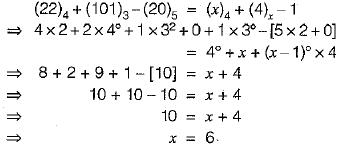QUESTION: 2

### If (28)x in base x number system is equal to (37)y in base y number system, the possible values of x and y are:

Solution: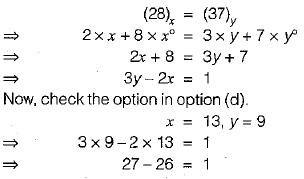QUESTION: 3

### Suppose the largest n-bit binary number requires ‘d’ digits in decimal representation. Which of the following relation between ‘n’ and 'd’ is approximately correct?

Solution: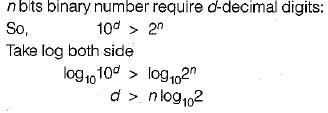QUESTION: 4

Let A = 1111 1010 and B = 0000 1010 be two 8-bit 2’s complement numbers. Their product in 2’s complement is

Solution:

A = 11111010
Since MSB is 1 hence number is negative i.e. - (00000110)
= -(00000110)
A = - 6
B = 00001010,
There is number 2’s complement representation for positive number B = +10
A x B = 10 x (- 6)
= - 60
Binary representation of - 60 = 00111100
Now take 2’s complement = 11000100

QUESTION: 5

Which of the following 4-bit numbers equals its 1’s complement?

Solution:

Since in 1 ’s complete every bits get complemented so, no such number exist whose complement is same while in 2’s complement 8 and 0 are exist.

QUESTION: 6

If the decimal number is 3.248 x 104 then its 32 bit equivalent floating number is

Solution:

Decimal number 3.248 x 104
So sign bit should be 0 since number is positive.
3.248 x 104 = 32480
= 1111110111000002
= 1.11111011100000 x 214
In normalized representation:
Biased = 14 + 127 = 14
= 100011012
So, number is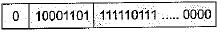QUESTION: 7

A computer uses 8 digit mantissa and 2 digit exponent. If a = 0.052 and b = 28 E + 11, then b + a - b will

Solution:

a = 0.052 and b = 28 E + 1
So, b + a - b will b
a = 0.52 exponent
= -1
b = 28 E + 11 mantissa
= 0,28 exponent
= 13
b + a = 28000000000000 E - 1 + 0.52 E-1
= 280000000000.52 E - 1
With is equal to 28 E + 11
So, b - b = 0

QUESTION: 8

Consider the following floating point format;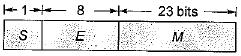S : Sign bit
E : Exponent
M : Mantissa
The value of floating point number in this system is, V = (-1 )s x 2E-127 x 1.F
Then what is the corresponding decimal value if the floating point stored is: 3F800000

Solution: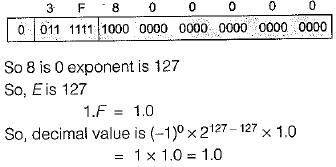QUESTION: 9

A single-precision floating point number contains the sequence of bits 100011111 00000000001 000000000000, Information is stored in the following left-to-right order, sign bit, exponent (bias 127) and mantissa. Which of the following representation in decimal is equivalent?

Solution: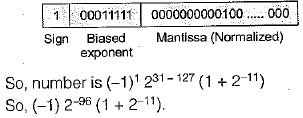QUESTION: 10

Sign extension is a step in

Solution:

Sign extension is the operation, in computer arithmetic, of increasing the number of bits of a binary number while preserving the number’s sign (positive/negative) and value.

QUESTION: 11

The decimal number 0.239 x 213 has the following hexadecimal representation (without normalization and rounding off:

Solution:

The decimal number is 0.239 x 213
We have to find hexadecimal representation without normalization.
Biased exponent = 13 + 64 = 77
Representing 77 in binary (77)10 = (1001101)2
Representing mantissa in binary
senting mantissa in binary (0.239)10 = 0.00111101000101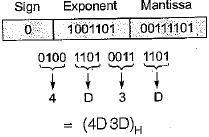QUESTION: 12

The vales of x and y, if (x567)8 + (2yx5)8 = (71 yx)8 is

Solution:

(x 567)8
(2yx5)8
(71yx)8
x will be 4 and y will be 3.

QUESTION: 13

A decimal number has 25 digits. The numberof bits needed for its equivalent binary representation is, approximately,

Solution: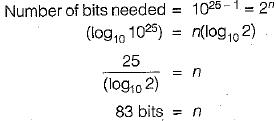QUESTION: 14

The greatest negative number which can be stored in computer that has 8-bit work length and uses 2's complement arithmetic is

Solution:

Smallest number stored in 8 bit 2’s complement arithm etic is -2n-1
=-28- 1
= -128

QUESTION: 15

In the given network of AND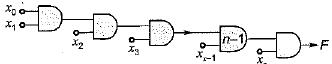‘F' will be written as:

Solution:

F will represents x0 x1 x2 .... xn-1 xn.